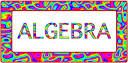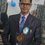# ALGEBRAAlgebra is one of the broad parts of mathematics, together with number theory, geometry and analysis. Algebra arose from the idea that one can perform operations of arithmetic with non-numerical mathematical objects. At the beginning of algebra, and at elementary level, these objects are variables representing either numbers that are not yet known (unknowns) or unspecified numbers (indeterminates or parameters). This allows one to state and prove properties that are true no matter which specific numbers are involved. More generally, these objects may have various basic properties, and, presently, algebra is divided in several subareas which include linear algebra, group theory, ring theory and combinatorics (see below for more subareas). Elementary algebra is the part of algebra that is usually taught in elementary courses of mathematics. Abstract algebra is a name usually given to the study of the algebraic structures (such as groups, rings, fields and algebras) themselves. Algebra is also the name of various specific mathematical structures occurring in algebra. To distinguish between the meanings of the word, see below. The adjective "algebraic" usually means relation to algebra, as in "algebraic structure". For historical reasons, it may also mean relation with the roots of polynomial equations, like in algebraic number, algebraic extension or algebraic expression. This comes from the fact that, until the end of 19th century, algebra was essentially the same area as the theory of equations. A witness of that is the fundamental theorem of algebra, which nowadays is not considered as belonging to algebra.Note by Akash Dhasmana
7 years, 4 months ago

This discussion board is a place to discuss our Daily Challenges and the math and science related to those challenges. Explanations are more than just a solution — they should explain the steps and thinking strategies that you used to obtain the solution. Comments should further the discussion of math and science.

When posting on Brilliant:

• Use the emojis to react to an explanation, whether you're congratulating a job well done , or just really confused .
• Ask specific questions about the challenge or the steps in somebody's explanation. Well-posed questions can add a lot to the discussion, but posting "I don't understand!" doesn't help anyone.
• Try to contribute something new to the discussion, whether it is an extension, generalization or other idea related to the challenge.
• Stay on topic — we're all here to learn more about math and science, not to hear about your favorite get-rich-quick scheme or current world events.

MarkdownAppears as
*italics* or _italics_ italics
**bold** or __bold__ bold
- bulleted- list
• bulleted
• list
1. numbered2. list
1. numbered
2. list
Note: you must add a full line of space before and after lists for them to show up correctly
paragraph 1paragraph 2

paragraph 1

paragraph 2

[example link](https://brilliant.org)example link
> This is a quote
This is a quote
    # I indented these lines
# 4 spaces, and now they show
# up as a code block.

print "hello world"
# I indented these lines
# 4 spaces, and now they show
# up as a code block.

print "hello world"
MathAppears as
Remember to wrap math in $$ ... $$ or $ ... $ to ensure proper formatting.
2 \times 3 $2 \times 3$
2^{34} $2^{34}$
a_{i-1} $a_{i-1}$
\frac{2}{3} $\frac{2}{3}$
\sqrt{2} $\sqrt{2}$
\sum_{i=1}^3 $\sum_{i=1}^3$
\sin \theta $\sin \theta$
\boxed{123} $\boxed{123}$

## Comments

Sort by:

Top Newest

algebra is very important

- 7 years, 4 months ago

Log in to reply

×

Problem Loading...

Note Loading...

Set Loading...